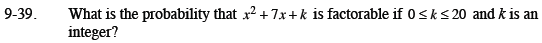Home > CCA2 > Chapter 9 > Lesson 9.1.3 > Problem9-39

9-39.

What is the probability that x2 + 7x + k is factorable if 0 ≤ k ≤ 20 and k is an integer? Homework Help ✎How many possible values are there for k? This is the denominator of the probability.

What numbers between 0 and 20 starting with 1 and going to 20 have two factors that add to 7? Note: 0 works because x would be a factor.

$\frac{\text{Number of }k\text{s that work}}{\text{All possible }k\text{s}}$